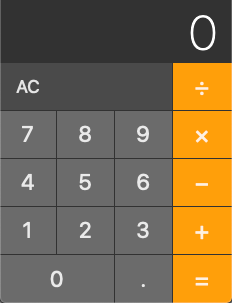This page is a work in progress.You can help improve it. →

# Calculator Fun

Practice is key to gaining the mileage needed to conquer programming. A very common practice problem is to recreate a working calculator. A basic calculator is a good exercise in using state, effects, and components to create a powerful and robust UI.

## Objectives

• Practice using functional components with hooks
• Practice implementing a UI wire-frame

## Requirements

Create a simple calculator that allows the user to add, multiply, subtract, and divide two numbers. Here is a sample UI:• Take this in small steps just like we did in class. Start with the HTML, then CSS, then with basic functionality.

### Setup

``degit \$GITHUB_USER/react-project-template ReactCalculator``

### Explorer Mode

• Implement something similar to the example UI using HTML and CSS inside a React app
• As a user, I should be able to add two numbers
• As a user, I should be able to add subtract numbers
• As a user, I should be able to add multiple numbers
• As a user, I should be able to add divide numbers
• The clear button should reset the current calculation

• Be able to chain together results. For example, the user should be able to input `2 + 3 - 4 + 5` and get the correct answer